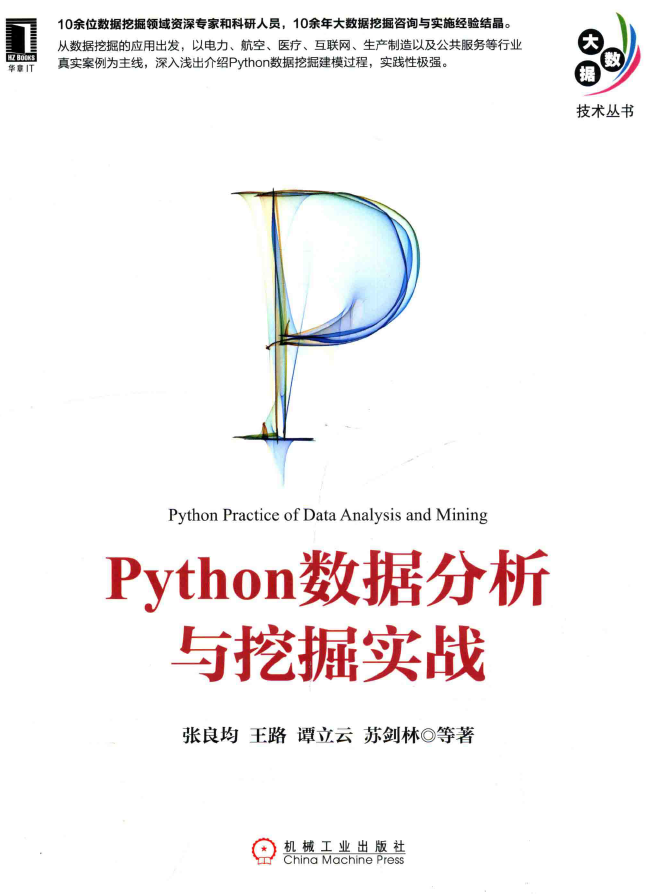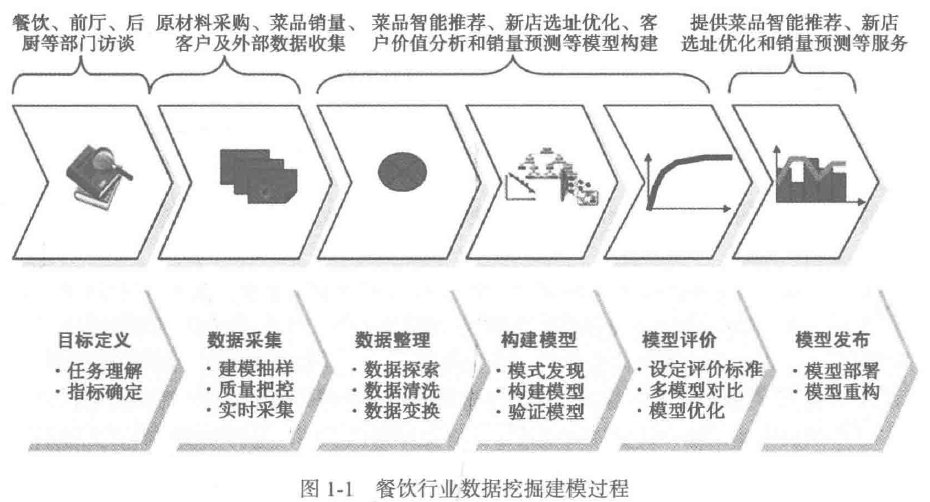2019-12-02 10:54:40 xazeal 阅读数 156
• ###### Python数据分析实战-Pandas

Pandas包是基于Python平台的数据管理利器，已经成为了Python进行数据分析和挖掘时的数据基础平台和事实上的工业标准，掌握其使用方法，是使用Python进行数据分析和数据挖掘的必备条件。        本课程通过讲解Pandas基础知识、DataFrame核心分析方法，以一系列实操案例使你快速掌握数据获取，数据清洗与整理，数据分析         pandas是在python最直接最流行的数据处理框架。可以这么说，如果你不会使用pandas，那你就谈不上会用python做数据分析。所以，pandas的重要性是不言而喻的，不管是想做简单的做数据分析和预测，还是想做高端的机器学习，深度学习，我们都必须学习pandas。  这门课程目标是，高效快速的让大家在最短的时间内掌握好pandas这个在python数据分析中不可缺少的数据分析框架。

480 人正在学习 去看看 李焱兵

### 树莓派python工业数据采集系统

*DAQM-4206–>CBT-1009—>树莓派

``````#!/usr/bin/env python
# -*- coding: utf_8 -*-
# DAQM-4206 测试程序,树莓派4B/3B....,CBT-1009
import serial
import modbus_tk
import modbus_tk.defines as cst
from modbus_tk import modbus_rtu
import time

def sleeptime(hour, min, sec):
return hour * 3600 + min * 60 + sec
second = sleeptime(0, 0, 1)
master = modbus_rtu.RtuMaster(
serial.Serial('/dev/ttyUSB0', baudrate=9600,

bytesize=8, parity='N', stopbits=1,xonxoff=0)
)
master.set_timeout(2.0)
master.set_verbose(True)

while True:

data = master.execute(1, cst.READ_HOLDING_REGISTERS, 256, 8)
time.sleep(second)
index = 0
temp= [0 for i in range(len(data ))]
for index in range(len(data )):
temp[index ] = data[index ]/4095.0*20.0
print(temp)    #打印结果

DAQM-4206采集工业4~20MA信号，
CBT-1009是隔离型USB转485

[15.789987789987789, 0.0, 0.0, 0.0, 0.0, 0.0, 0.0, 0.0]
[15.789987789987789, 0.0, 0.0, 0.0, 0.0, 0.0, 0.0, 0.0]
[15.789987789987789, 0.0, 0.0, 0.0, 0.0, 0.0, 0.0, 0.0]
[15.789987789987789, 0.0, 0.0, 0.0, 0.0, 0.0, 0.0, 0.0]
[15.789987789987789, 0.0, 0.0, 0.0, 0.0, 0.0, 0.0, 0.0]
[15.789987789987789, 0.0, 0.0, 0.0, 0.0, 0.0, 0.0, 0.0]
[15.794871794871794, 0.0, 0.0, 0.0, 0.0, 0.0, 0.0, 0.0]
[15.789987789987789, 0.0, 0.0, 0.0, 0.0, 0.0, 0.0, 0.0]
[15.789987789987789, 0.0, 0.0, 0.0, 0.0, 0.0, 0.0, 0.0]
[15.789987789987789, 0.0, 0.0, 0.0, 0.0, 0.0, 0.0, 0.0]
[15.789987789987789, 0.0, 0.0, 0.0, 0.0, 0.0, 0.0, 0.0]
[15.789987789987789, 0.0, 0.0, 0.0, 0.0, 0.0, 0.0, 0.0]
[15.794871794871794, 0.0, 0.0, 0.0, 0.0, 0.0, 0.0, 0.0]
[15.789987789987789, 0.0, 0.0, 0.0, 0.0, 0.0, 0.0, 0.0]
[15.789987789987789, 0.0, 0.0, 0.0, 0.0, 0.0, 0.0, 0.0]
[15.789987789987789, 0.0, 0.0, 0.0, 0.0, 0.0, 0.0, 0.0]
[15.789987789987789, 0.0, 0.0, 0.0, 0.0, 0.0, 0.0, 0.0]
[15.789987789987789, 0.0, 0.0, 0.0, 0.0, 0.0, 0.0, 0.0]
[15.794871794871794, 0.0, 0.0, 0.0, 0.0, 0.0, 0.0, 0.0]
[15.794871794871794, 0.0, 0.0, 0.0, 0.0, 0.0, 0.0, 0.0]
[15.794871794871794, 0.0, 0.0, 0.0, 0.0, 0.0, 0.0, 0.0]
[15.794871794871794, 0.0, 0.0, 0.0, 0.0, 0.0, 0.0, 0.0]
[15.794871794871794, 0.0, 0.0, 0.0, 0.0, 0.0, 0.0, 0.0]

``````

python 工业大数据 相关内容

2019-03-30 11:15:50 qq_42642945 阅读数 232
• ###### Python数据分析实战-Pandas

Pandas包是基于Python平台的数据管理利器，已经成为了Python进行数据分析和挖掘时的数据基础平台和事实上的工业标准，掌握其使用方法，是使用Python进行数据分析和数据挖掘的必备条件。        本课程通过讲解Pandas基础知识、DataFrame核心分析方法，以一系列实操案例使你快速掌握数据获取，数据清洗与整理，数据分析         pandas是在python最直接最流行的数据处理框架。可以这么说，如果你不会使用pandas，那你就谈不上会用python做数据分析。所以，pandas的重要性是不言而喻的，不管是想做简单的做数据分析和预测，还是想做高端的机器学习，深度学习，我们都必须学习pandas。  这门课程目标是，高效快速的让大家在最短的时间内掌握好pandas这个在python数据分析中不可缺少的数据分析框架。

480 人正在学习 去看看 李焱兵

### 文章目录# 第一章数据挖掘基础

## 2.数据挖掘建模过程

• 目标定义

• 任务理解
• 指标确定
• 数据采集

• 建模抽样
• 质量把控
• 实时采集
• 数据整理

• 数据探索
• 数据清洗
• 数据变换
• 构建模型

• 模式发现
• 构建模型
• 验证模型
• 模型评价

• 设定评价标准
• 多模型对比
• 模型优化
• 模型发布

• 模型部署
• 模型重构### 数据抽样

• 相关性
• 可靠性
• 有效性

• 资料完整无缺，各类指标项齐全。
• 数据准确无误，反映的都是正常状态下的水平

• 随机抽样
• 等距抽样
• 分层抽样
• 从起始顺序抽样
• 分类抽样

• 异常值分析
• 缺失值分析
• 相关性分析
• 周期性分析

• 数据筛选
• 数据变量转换
• 缺失值处理
• 坏数据处理
• 数据标准化
• 主成分分析
• 属性选择
• 数据违约

## 3. 常见的数据挖掘建模工具

• SAS
• SPSS
• SQL
• PYTHON
• WEKA
• KNIME
• RapidMine
• TipDM

python 工业大数据 相关内容

2018-06-09 12:48:25 qq_39407518 阅读数 671
• ###### Python数据分析实战-Pandas

Pandas包是基于Python平台的数据管理利器，已经成为了Python进行数据分析和挖掘时的数据基础平台和事实上的工业标准，掌握其使用方法，是使用Python进行数据分析和数据挖掘的必备条件。        本课程通过讲解Pandas基础知识、DataFrame核心分析方法，以一系列实操案例使你快速掌握数据获取，数据清洗与整理，数据分析         pandas是在python最直接最流行的数据处理框架。可以这么说，如果你不会使用pandas，那你就谈不上会用python做数据分析。所以，pandas的重要性是不言而喻的，不管是想做简单的做数据分析和预测，还是想做高端的机器学习，深度学习，我们都必须学习pandas。  这门课程目标是，高效快速的让大家在最短的时间内掌握好pandas这个在python数据分析中不可缺少的数据分析框架。

480 人正在学习 去看看 李焱兵

### 一、csv数据处理

csv表示”Comma-Separated Values(逗号分隔的值)”，csv文件时简化的电子表格，保存为纯文本文件。
python中解析csv文件可以使用csv模块。

 ```1 2 3 4 5 6 ``` ```import csv with open("example.csv", encoding='utf-8') as dataObject: dataReader = csv.reader(dataObject) for row in dataReader: print("第{0}行:{1}".format(dataReader.line_num, row)) ```

Reader对象的`line_num`变量是当前的行号。

 ```1 2 3 ``` ```第1行:['2018/06/09', 'Apples', '73'] 第2行:['2018/06/10', 'Cherries', '85'] 第3行:['2018/06/11', 'Pears', '14'] ```

#### Writer

Write对象可以将数据写入到csv文件中。

 ```1 2 3 4 5 6 7 ``` ```import csv with open("test.csv", 'w', encoding='utf-8', newline='') as outputFile: dataWriter = csv.writer(outputFile) dataWriter.writerow(['a', 'b', 'c']) dataWriter.writerow(['啊', '吧', '次']) dataWriter.writerow(['A', 'Hello,World!', 'C']) ```

 ```1 2 3 ``` ```a,b,c 啊,吧,次 A,"Hello,World!",C ```

• delimiter 指定分隔符，默认为逗号
• lineterminator 行距，默认为单倍行距 ```1 ``` ```dataWriter = csv.writer(outputFile, delimiter='\t', lineterminator='\n\n') ```

### 二、excel数据处理

``python提供有第三方库来支持对excel的操作，python处理excel文件用的第三方模块库有xlrd、xlwt、xluntils和pyExcelerator，除此之外，python处理excel还可以用win32com和openpyxl模块。下面我们先安装第三方库：``
``````Pip install xlrd
Pip install xlwt
Pip install xluntils
Pip install pyExcelerator
``````

Xlrd只能进行读取excel文件，没法进行写入文件，xlwt可以写入文件，但是不能在已有的excel的文件上进行修改，如果有这个需求，就需要使用xluntils模块了，pyExcelerator模块与xlwt类似，也可以用来生成excel文件。

#### 2.1读取单表文件：

``````import xlrd

data = xlrd.open_workbook('test.xlsx')
table = data.sheets()    # 打开第一张表
nrows = table.nrows     # 获取表的行数
for i in range(nrows):  # 循环逐行打印
print(table.row_values(i))      #通过row_values来获取每行的值

``````

#### 2.2 读取单表文件复杂例子：

``````# 打开一个workbook
workbook = xlrd.open_workbook('testdata.xlsx')
# 抓取所有sheet页的名称
worksheets = workbook.sheet_names()
print(workbook.sheets())
print('worksheets is {0}'.format(worksheets))
# 定位到sheet1
# worksheet1 = workbook.sheet_by_name(u'Sheet1')
worksheet1 = workbook.sheets()
"""
#通过索引顺序获取
worksheet1 = workbook.sheets()
"""
"""
#遍历所有sheet对象
for worksheet_name in worksheets:
worksheet = workbook.sheet_by_name(worksheet_name)
"""
# 遍历sheet1中所有行row
num_rows = worksheet1.nrows
for curr_row in range(num_rows):
row = worksheet1.row_values(curr_row)
print('row%s is %s' % (curr_row, row))
# 遍历sheet1中所有列col
num_cols = worksheet1.ncols
for curr_col in range(num_cols):
col = worksheet1.col_values(curr_col)
print('col%s is %s' % (curr_col, col))
# 遍历sheet1中所有单元格cell
for rown in range(num_rows):
for coln in range(num_cols):
cell = worksheet1.cell_value(rown, coln)
print(cell)
``````

#### 2.3、写入excel文件

``````import xlwt
#创建workbook和sheet对象
workbook = xlwt.Workbook() #注意Workbook的开头W要大写
#向sheet页中写入数据
sheet1.write(0,0,'this should overwrite1')
sheet1.write(0,1,'aaaaaaaaaaaa')
sheet2.write(0,0,'this should overwrite2')
sheet2.write(1,2,'bbbbbbbbbbbbb')

#-----------使用样式-----------------------------------
#初始化样式
style = xlwt.XFStyle()
#为样式创建字体
font = xlwt.Font()
font.name = 'Times New Roman'
font.bold = True
#设置样式的字体
style.font = font
#使用样式
sheet3.write(0,1,'some bold Times text',style)

#保存该excel文件,有同名文件时直接覆盖
workbook.save('test2.xls')
print('创建excel文件完成!')
``````

#### 2.4、Excele来处理超链接

``````import codecs
import xlwt
book = xlwt.Workbook()
line=0
for i in range(9):
line += 1
book.save('simple2.xls')
for i in range(0, 9):
file = str(i) + ".txt"
with codecs.open(file, 'w') as f:
f.write(str(i)*10)
``````

#### 2.5、Python进行修改excel文件：

``````import xlrd
import xlutils.copy
#打开一个workbook
rb = xlrd.open_workbook('aaa111.xls')
wb = xlutils.copy.copy(rb)
#获取sheet对象，通过sheet_by_index()获取的sheet对象没有write()方法
ws = wb.get_sheet(0)
#写入数据
ws.write(10, 10, 'changed!')
#添加sheet页
#利用保存时同名覆盖达到修改excel文件的目的,注意未被修改的内容保持不变
wb.save('aaa111.xls')``````

python 工业大数据 相关内容

2019-11-08 15:48:54 fcy11 阅读数 50
• ###### Python数据分析实战-Pandas

Pandas包是基于Python平台的数据管理利器，已经成为了Python进行数据分析和挖掘时的数据基础平台和事实上的工业标准，掌握其使用方法，是使用Python进行数据分析和数据挖掘的必备条件。        本课程通过讲解Pandas基础知识、DataFrame核心分析方法，以一系列实操案例使你快速掌握数据获取，数据清洗与整理，数据分析         pandas是在python最直接最流行的数据处理框架。可以这么说，如果你不会使用pandas，那你就谈不上会用python做数据分析。所以，pandas的重要性是不言而喻的，不管是想做简单的做数据分析和预测，还是想做高端的机器学习，深度学习，我们都必须学习pandas。  这门课程目标是，高效快速的让大家在最短的时间内掌握好pandas这个在python数据分析中不可缺少的数据分析框架。

480 人正在学习 去看看 李焱兵

• 数据整合（不同来源数据统一形式，时间对齐）
• 特征选择
• 数据清洗（坏点剔除、噪声滤波、缺失值处理）

# 数据整合

``````from scipy import interpolate
import pandas as pd
import numpy as np

def interpolation():
# 日期转化成秒
x = self.indoor_temp_filter_dic[key][:, 0]
y = self.indoor_temp_filter_dic[key][:, 2]
x1 = []
re_date = []
for i in x:
i = pd.Timestamp(i)
i = i.timestamp()
x1.append(i)

# 截取时间范围2018-12-22 00:00:00 - 2019-03-18 00:00:00, 间隔per = 5min, 转化成秒
start = pd.Timestamp('2018-12-22 00:00:00')
start1 = start.timestamp()
end = pd.Timestamp('2019-03-18 00:00:00')
end1 = end.timestamp()
interval = pd.Timedelta(minutes=5)
interval1 = interval.seconds

per = (end1 - start1)/interval1
kk = np.linspace(start1, end1, per+1)

f = interpolate.interp1d(x1, y, kind='linear')
ynew = f(kk)

# 再将以秒为单位的数据转化回Timestamp类型保存
for k in kk:
k = pd.Timestamp(k, unit='s')
re_date.append(k)

self.indoor_temp_interpolation_dic[key] = np.column_stack((re_date, ynew))
``````

# 特征提取

``````def Heatmap(dataset):
key_list = ['indoor_temp','water_supply_temp','outdoor_temp','wind_pow','wind_air','light_time','humidity','air_pre']
dataset = np.array(dataset)
cor_matri = np.corrcoef(dataset.tolist(), rowvar=0)
sns.heatmap(pd.DataFrame(cor_matri, columns=key_list, index=key_list), annot=True, vmax=1, vmin=0,
xticklabels=True,
yticklabels=True, square=True, cmap="YlGnBu")
fig, ax = plt.subplots(figsize=(8, 8))
ax.set_title(' Heat Map ', fontsize=18)
ax.set_ylabel('Y', fontsize=18)
ax.set_xlabel('X', fontsize=18)
plt.show()
plt.savefig('Random.png')
``````

``````from scipy.stats import pearsonr

p = pearsonr(x, y)
``````

# 异常值清洗

``````# input: 一维数组
# per：相邻几个点取平均
def Filter(inputs, per):
temp = []
lengh = len(inputs)
for i, element in enumerate(inputs):
if (i >= int(per/2)) and (i <= lengh-int(per/2)-1):
filter_list = inputs[i-int(per/2) : i + int(per/2) + 1]
temp1 = [sum(filter_list) / per]
else:
temp1 = [inputs[i]]
temp.append(temp1)
return temp
``````

• 观察数据直接剔除——根据阈值、相邻点变化量等
• 正态分布3δ原则，剔除小概率事件
• 通过极差和四分位数间距检测
• 聚类（基于距离、密度）

# 随机生产训练集测试集

``````	proportion = 4/5
dataNumber = dataSet.__len__()  # 数据集数据条数
index = int(dataNumber * proportion)  # 训练集数据条数
testDataSet = []  # 测试数据集
trainDataSet = []  # 训练数据集

indexes = np.array(range(0, dataNumber))
random.shuffle(indexes)
train_indexes = indexes[0:index]
test_indexes = indexes[index: dataNumber]

trainDataSet = np.array(dataSet)[train_indexes, :]
testDataSet = np.array(dataSet)[test_indexes, :]
``````

python 工业大数据 相关内容